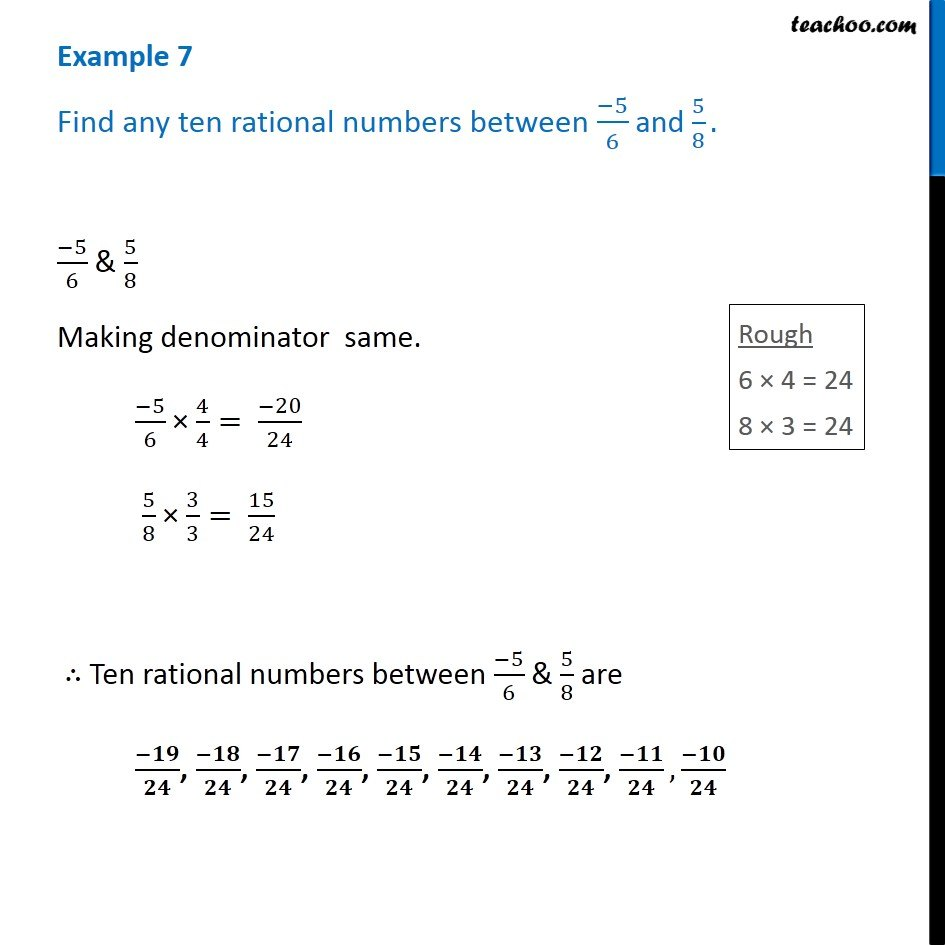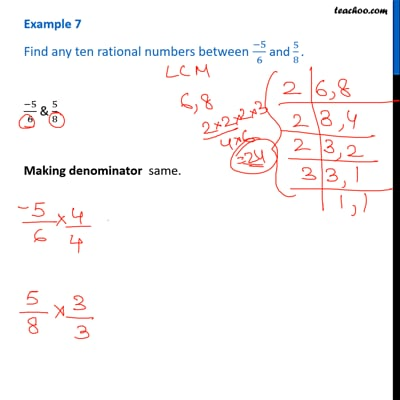Examples

Chapter 1 Class 8 Rational Numbers
Serial order wiseThis video is only available for Teachoo black users

Learn in your speed, with individual attention - Teachoo Maths 1-on-1 Class

### Transcript

Question 4 Find any ten rational numbers between (−5)/6 and 5/8. (−5)/6 & 5/8 Making denominator same. (−5)/6 × 4/4= (−20)/24 5/8 × 3/3= 15/24 ∴ Ten rational numbers between (−5)/6 & 5/8 are (−𝟏𝟗)/𝟐𝟒, (−𝟏𝟖)/𝟐𝟒, (−𝟏𝟕)/𝟐𝟒, (−𝟏𝟔)/𝟐𝟒, (−𝟏𝟓)/𝟐𝟒, (−𝟏𝟒)/𝟐𝟒, (−𝟏𝟑)/𝟐𝟒, (−𝟏𝟐)/𝟐𝟒, (−𝟏𝟏)/𝟐𝟒,(−𝟏𝟎)/𝟐𝟒